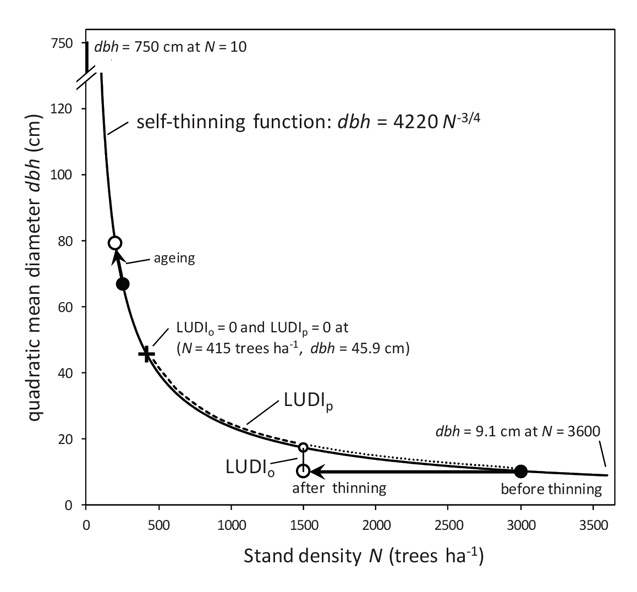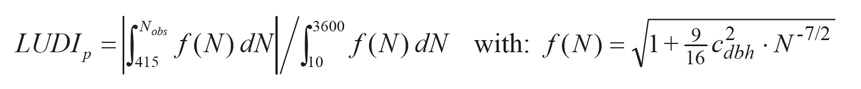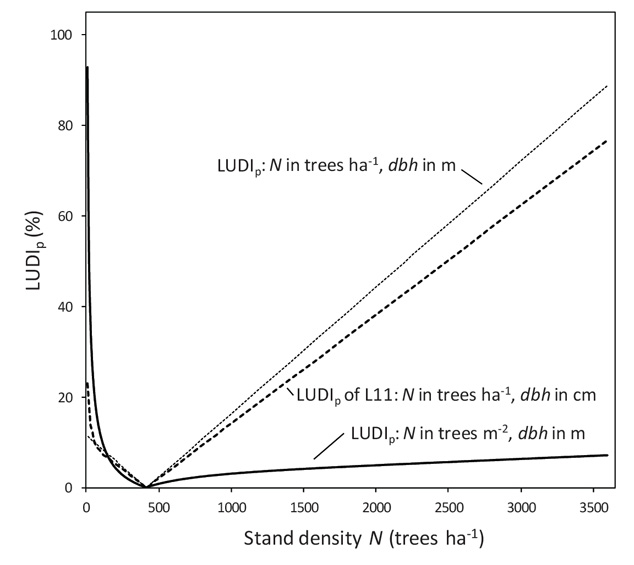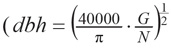Ecological Archives A023-032-A1

Peter Schall, Christian Ammer. 2013. Can land use intensity be reliably quantified by using a single self-thinning relationship?. Ecological Applications 23:675–677. http://dx.doi.org/10.1890/12-0847.1

Appendix A. Conceptual shortcomings and inaccuracies in the inventory data used by L11.

In this appendix we use the definition of the terms N, Nobs, Nmaxx, Npr, cdbh, dbh, dbhobs, dbhmax, dbhmaxx, dbhmaxxobs, LUDIo, LUDIp, LUDIppr given in L11. Readers may refer to this paper for definitions.

Conceptual shortcomings

LUDI is supposed to measure “the difference between potential and actual biomass storage” at “long and short timescales associated with management and disturbance” (by components LUDIp, the “planning intensity”) and LUDIo, the “operational intensity”. However, the components of LUDI (Fig. A1) definitely fail do not measure the difference between potential and actual biomass storage:

(i) The mismatch is obvious for LUDIo, which is defined in L11 as the relative deviation of the observed quadratic mean diameter at breast height (dbhobs) from the corresponding maximum dbh indicated by the self-thinning function (dbhmax) for the observed stand density Nobs. As LUDIo relates linearly to diameter - not to biomass - a value of, e.g., 0.5 (i.e., dbhobs is half of dbhmax for Nobs) actually means that observed biomass is 0.19 (= 0.52.4) of the potential biomass of a fully stocked stand for a given tree number, since tree biomass scales to dbh to the power of ~2.4 (Djomo et al. 2010, Zianis and Mencuccini 2004).FIG. A1. Conceptual figure of land use and disturbance intensity LUDI of L11 with components LUDIp “planning intensity” and LUDIo “operational intensity” showing reference, minimum and maximum stand density N and the corresponding diameters dbh under self-thinning. Note that dbh = 750 cm for N = 10 trees/ha and not 520 cm, as mistakenly given in Fig. 1 of L11.

(ii) For LUDIp the mismatch is masked in L11 by a definition that lacks a physical meaning, since it is based on the “length” of segments of the self-thinning function in the diameter-stand density space. In order to clarify the meaning of LUDIp we present here the mathematical definition for the self-thinning function dbh = cdbh·N-3/4, which was not specified in L11:Function f(N) describes the length of line segments along the self-thinning function for infinitesimal changes in N (Fig. A1). The value of the proportionality constant cdbh is subject to units of measure of N and dbh: cdbh = 4220 for N (trees/ha), dbh (cm); cdbh = 42.2 for N (trees/ha), dbh (m); cdbh = 0.0422 for N (trees/m²), dbh (m). The sensitivity of the LUDIp equation to units of measure is evident. This property of LUDIp, which is generally inappropriate for a scientific measure, is due to the fact that the “length” of line segments of the self-thinning function in the diameter-density space has no physical meaning. Instead, LUDIp is controlled by N and dbh, dependent on the scaling of the variables, i.e., the actual numerical contribution of a variable to the euclidean distance (Fig A2). Only if dbh and N are specified in centimeters and trees per hectare, LUDIp behaves as described in Appendix B of L11. Then LUDIp is almost exclusively controlled by stand density if N > 100 trees/ha. However, this setting is arbitrary. If for example basic units are used to describe dbh (m) and N (trees/m²) the picture changes completely (Fig. A2); then LUDIp is controlled by the deviation in diameter from reference. Thus, the densest stands considered in L11 (3600 trees/ha) are characterized by LUDIp = 7.1% if using basic units compared to 76.7% if using centimeters and hectares (Fig. A2).FIG. A2. LUDIp as defined in L11 is sensitive to the units used to characterize stand density N and mean diameter dbh. Values for LUDIp are exemplarily given for three combinations of units of measure of N (trees/ha vs. trees/m²) and dbh (cm vs. m).

In summary, LUDIp as defined by L11 is scientifically inaccurate and, when calculated with the combination of units arbitrarily used in L11, almost exclusively measures the deviation of actual stand density Nobs from the reference stand density Nref irrespective of diameter (Fig. A2).

(iii) The index LUDI, defined by L11 as the sum of LUDIo and LUDIp, thus combines a relative deviation in dbh for a given N and the relative deviation of N from a reference stand density irrespective of dbh. Unlike suggested by L11, neither LUDI nor its components relate to biomass storage or specific timescales. In our opinion the authors ignored the only interpretation of the self-thinning relationship that makes sense in terms of biomass storage: namely the difference between actual N and maximum N for a given dbh, which is linearly related to the deficit in standing biomass. According to the approach of L11, LUDI is lowest - signaling minimum intensity - for a forest that comprises 415 trees/ha (LUDIp = 0) with mean diameter 45.9 cm (LUDIo = 0), irrespective of tree species identity and site quality (Fig. A1). Any thinning or disturbance that does not reduce stand density below 415 trees/ha decreases “planning intensity” LUDIp while stand age related density changes increase “planning intensity” afterwards. The thinning depicted in Fig. A1, affecting 50% of trees (and biomass), is measured by LUDI as intensity change from 62.3% (N = 3000 trees/ha, dbh = 10.4 cm) to 66.7% (N = 1500 trees/ha, dbh = 10.4 cm). The biomass accumulation with aging, depicted in Fig. A1, is measured by LUDI as intensity increase from 4.1% (N = 250 trees/ha, dbh = 67.1 cm) to 5.3% (N = 200 trees/ha, dbh = 79.3 cm).

In summary, LUDI fails to quantify the intensity of a thinning or disturbance (Fig. A1 and Fig. A2), but at least the response direction is correct. If stands accumulate biomass through ageing, even the direction of response is questionable given that stand density falls below 415 trees/ha (Fig. A2).

Inconsistency between results and methods

With respect to the summary characteristics of the plots of pristine forests in Slovakia (L11-Appendix E), we found:

• The average stem number N for total plots (i.e., Npr) is not 415 trees/ha but 442 trees/ha.
• The number of plots should be 35 for total plots, and 33 for Fagus-Picea plots instead of 45, and 43 respectively.
• The average dbh of total plots should be 36.3 cm instead of 39 cm.
• The mean and standard deviation values for basal area, N, dbh, and wood volume of Picea and Fagus-Picea pristine forest stand-type plots could be clarified (Table A1).

The values provided for total plots are often mathematically inaccurate. This inaccuracy affects the results as the average stem number of pristine forests Npr serves as reference for LUDIp of managed forests (Eq. 4 and Fig. 1b, L11). We assume that the incorrect value (i.e., 415 trees/ha) was used in calculations because it is provided in L11-Appendix B (Procedure for the calculation of the distance along the self-thinning line).

With respect to values for individual pristine forest plots in Slovakia (L11-Appendix E) we found that:

• All values for dbh, (i.e., quadratic mean diameter with G denoting basal area in m²/ha and N stem number in trees/ha) were calculated using a value of about 3.41 (three point four one) instead of 3.14 to represent π.
• The values cited by Drößler (2006) for Kyjov and Hovesova forests are not reported correctly, although the correct values are explicitly stated by Dößler (basal area: p. 18, N: p. 19, dbh: p. 21, wood volume: p. 16). Values for basal area (m²/ha), N (trees/ha), dbh (cm) and wood volume (m3/ha) should read for Kyjov 32, 320, 35.4, and 451 instead of 35.35, 252, 40.56, and 500, and for Hovesova 35, 290, 38.8, and 716 instead of 40, 193, 49.31, and 870 respectively. The dbh reported in L11 is incorrect for two reasons: due, firstly, to the incorrect citation of basal area and N and, secondly, to the incorrect value of π used.
• 9 values cited from Korpel’ (1995) are reported incorrectly.
• The weighting given to the two studies from which information on composition of pristine forests was acquired - Korpel’ (1995) and Drößler (2006) - was imbalanced. Two of the 35 samples used in L11 were obtained from Drößler (2006). These two samples represented a sample area of 7.81 ha for Kyjov, and 8.60 ha for Hovesova forests (on average: 8.2 ha) because Drößler (2006, p. 6) followed a systematic sampling design using 20 plots for Kyjov and 22 plots for Hovesova forest, with each plot 62.5m × 62.5m in size. It remains unclear to us whether L11 used total forest means or means for selected plots, which may explain the discrepancies outlined above.
The remaining 33 samples taken from Korpel’ (1995) represent an average sampling area of 0.067 ha (1.33 ha / 20 plots), as Korpel’ used plot sizes of 10m × 50m (1 plot), 10m × 60m (6 plots), 10m × 70m (12 plots) and 10m × 80m (1 plot). For 13 of these 20 plots, data collected at two points in time were seen to represent independent samples. With respect to the area sampled to obtain each value provided in L11-Appendix E, Drößler’s (2006) study was weighted by the factor 0.0081 (0.067 ha / 8.2 ha) relative to the sample from Korpel’s (1995) work, even when the pseudo-replicated sample data in Korpel’ (1995) was not taken into account.

The findings suggest that the state-of-the-art study by Drößler (2006), in which pristine forests were systematically sampled using replicates and an adequate plot size (cf. Drößler, 2006 and references therein), was attributed less value than data from Korpel’ (1995).

Table A1. Data from "Ecological Archives A021-146-A5" (Appendix E, Table E1) for pristine forests with LUDIo computed using equations 3 and 5 (cdbh = 4220, page 3277) and LUDIppr, which is LUDIp for pristine forests, computed using equations 4, 6 and 7 in Luyssaert et al. (2011).

 original data calculated using: dbhmax = Nobs^(-3/4) * 4220 A = (Nmaxx: 108 stems/ha, dbhmaxx: 126 cm) Species Location Plot N = Nobs (trees/ha) basal area (m²/ha) dbh =dbhobs (cm) wood volume (m3/ha) dbhmax (cm) LUDIo dAB LUDIppr (dmax = 4152) Fagus/ Sitno DVF1 658 41.80 27.30 605 32.5 16.0 565.3 13.6 Hardwood/ DVF2 276 42.90 42.70 661 62.3 31.5 181.9 4.4 Abies DVF3 1026 41.20 21.70 530 23.3 6.8 933.4 22.5 DVF1 524 36.70 28.66 547 38.5 25.6 431.1 10.4 DVF2 294 37.30 38.58 607 59.4 35.1 200.1 4.8 DVF3 850 41.30 23.87 615 26.8 11.0 757.4 18.2 Fagus Kyjov Drößler 252 35.35 40.56 500 66.7 39.2 157.5 3.8 Fagus Kyjov DVF1 384 29.04 29.78 405 48.6 38.8 290.8 7.0 DVF2 644 35.40 25.39 447 33.0 23.1 551.3 13.3 DVF3 398 32.34 30.87 448 47.4 34.8 304.8 7.3 DVF1 360 34.01 33.29 455 51.1 34.8 266.6 6.4 DVF2 382 33.72 32.18 456 48.8 34.1 288.7 7.0 DVF3 350 34.84 34.17 512 52.2 34.5 256.6 6.2 Fagus Rozok DVF1 298 37.75 38.55 776 58.8 34.5 204.1 4.9 DVF2 292 44.32 42.20 794 59.7 29.4 198.1 4.8 DVF3 310 32.61 35.13 577 57.1 38.5 216.3 5.2 DVF1 197 33.03 44.35 709 80.3 44.7 100.8 2.4 DVF2 254 46.42 46.30 914 66.3 30.2 159.5 3.8 DVF3 332 34.63 34.98 709 54.3 35.5 238.5 5.7 Fagus Hovesova Drößler 193 40.00 49.31 870 81.5 39.5 96.6 2.3 Fagus Hovesova DVF1 222 32.40 41.38 655 73.4 43.6 126.7 3.1 DVF2 236 32.50 40.19 687 70.1 42.7 141.1 3.4 DVF3 214 35.60 44.17 773 75.4 41.4 118.5 2.9 Fagus/Abies Badin DVF1 436 40.80 33.13 745 44.2 25.1 342.9 8.3 Fagus/Abies Dobrov DVF3 358 71.00 48.23 1066 51.3 5.9 264.6 6.4 Fagus/Picea Kotlov DVF1 548 52.41 33.49 475 37.3 10.1 455.2 11.0 DVF2 868 71.36 31.05 697 26.4 -17.7 775.4 18.7 DVF3 806 50.71 27.17 500 27.9 2.6 713.3 17.2 DVF4 466 68.30 41.46 838 42.1 1.5 373.0 9.0 DVF1 386 44.52 36.78 449 48.5 24.1 292.8 7.1 DVF2 698 65.54 33.19 742 31.1 -6.8 605.3 14.6 DVF3 944 49.63 24.83 495 24.8 -0.2 851.4 20.5 DVF4 368 53.77 41.40 737 50.2 17.6 274.7 6.6 Picea Kosodrevina DVF3 376 65.00 45.03 805 49.4 8.9 282.7 6.8 Picea Polana DVF2 270 56.00 49.32 670 63.4 22.2 175.8 4.2 Result of recalculation of Table 2 from Luyssaert et al. (2011, page 3278) ) for pristine forests based on the values for plots shown above (confirmed values are printed in bold) Species # plots N (trees/ha) basal area (m²/ ha) dbh (cm) wood (m3/ha) dbhmax (cm) LUDIo LUDIppr total 35 mean 442.0 43.8 36.3 642.0 50.4 24.0 8.4 SD 228.6 12.2 7.6 158.0 16.3 16.1 5.5 Fagus-Picea 33 mean 449.2 42.8 35.6 636.2 50.0 24.5 8.6 SD 233.3 11.7 7.3 160.1 16.6 16.4 5.6 Picea 2 mean 323.0 60.5 47.2 737.5 56.4 15.5 5.5 SD 75.0 6.4 3.0 95.5 9.9 9.4 1.8

Literature Cited

Djomo, A. N., A. Ibrahima, J. Saborowski, und G. Gravenhorst. 2010. Allometric equations for biomass estimations in Cameroon and pan moist tropical equations including biomass data from Africa. Forest Ecology and Management 260:1873–1885.

Drössler, L. 2006. Struktur und Dynamik von zwei Buchenurwäldern in der Slovakei. University of Göttingen, Göttingen, Germany.

Korpel’, S. 1995. Die Urwälder der Westkarpaten. G. Fischer: Stuttgart, Jena, New York. 310 pp.

Luyssaert, S., D. Hessenmöller, N. von Lüpke, S. Kaiser, and E. D. Schulze. 2011. Quantifying land use and disturbance intensity in forestry, based on the self-thinning relationship. Ecological Applications 21:3271–3283.

Zianis, D., and M. Mencuccini. 2004. On simplifying allometric analyses of forest biomass. Forest Ecology and Management 187:311–332.